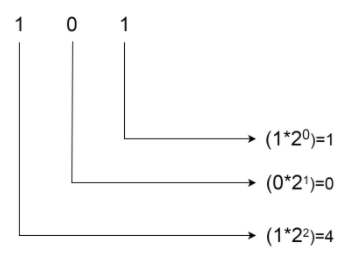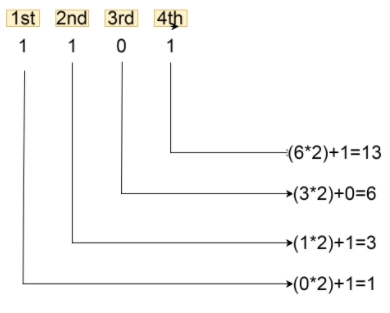New update is available. Click here to update.

# Binary to Decimal in C

## Introduction

Let’s say you went to a restaurant and had a good dinner. You paid and now you are supposed to rate the food on a scale from 0 to 10 and you rate it as 10. The adjacent restaurant also has a rating system, but it is from 0 to 5.  Someone rates the food there as 5. In both these cases, the food is tasty as the highest rating is given to both.

But at one place, it is 10 and at another, it is 5. So you see how the same things may have different representations in different systems. The same is the case with Number Systems. For example, 7 in the decimal number system is 111 in the binary number system.

Let's see how to convert binary numbers to decimals.

## Number System

A Number System is a way of representing values. There are different types of number systems, majorly binary, octal, decimal, and hexadecimal.

1. Binary Number System: It has numbers with base 2. For example, (1101)2, (1111)2, etc.
2. Octal Number System: It has numbers with base 8. For example, (347)8, (1324)8, etc.
3. Decimal Number System: It has numbers with base 10. For example, (590)10, (353)10, etc.
4. Hexadecimal Number System: It has numbers with base 16. For example, (16A)16, (D1)16, etc.

We can convert the values from one Number System to another with a set of rules. This article will discuss how to convert binary numbers to decimal numbers.

## Converting from Binary to Decimal in C

There are mainly two techniques to convert binary numbers to decimal numbers in C.
Let’s discuss them in detail.

### Approach 1

In this method, all the digits of the binary numbers are assigned some weight - starting from the rightmost, which is assigned a weight of zero(0). Moving towards the left, weight increases by one(1).

Now, every digit of the number is multiplied by 2 to power the weight of that digit. After this, all the products are added together and that is the decimal equivalent of it.
For example, let the binary number be (101)2. For this, the decimal equivalent will be calculated as follows:After adding all of them, 1+0+4=5. So, the decimal equivalent of (101)is 5.

#### Implementation in C

``````#include<stdio.h>
#include<math.h>
long long int Binary_To_Decimal(int val)
{
long long int ans=0;                //stores the decimal value
long long int mul=pow(2,0);         //stores power of 2
long long int num=val;
while(num)                          //calculating the product for every digit and adding to the result
{
int right=num%10;
num=num/10;
ans+=right*mul;
mul=mul*2;
}
return ans;
}
int main()
{
int n;
scanf("%d",&n);
printf("The decimal equivalent of binary number: %d is %lld",n,Binary_To_Decimal(n));
return 0;
}``````

Input 1:

``101``

Output 1:

``The decimal equivalent of binary number: 101 is 5``

Input 2:

``10111``

Output 2:

``The decimal equivalent of binary number: 10111 is 23``

Since the numbers, both binary and decimal, can be very large, we have used long long int data type. Of course, int can also be used. But, then, it might not work for large numbers. you can implement on an online c compiler.

### Approach 2

This method involves considering each digit of the binary number from the left, unlike the first method.
So, for every digit starting from the leftmost position, we add this digit to double the sum already obtained. Since there is no sum present for the leftmost digit, we start with 0. Let’s try to make it more clear with the help of an example.

Let the binary number be 1101.So, the decimal equivalent of 1101 is 13.

#### Implementation in C

``````#include<stdio.h>
#include<math.h>
long long int reverse_number(long long int num)   //reversing the number
{
long long ans=0;
while(num)
{
int rem=num%10;
ans=(ans*10)+rem;
num/=10;
}
return ans;
}
long long int Binary_To_Decimal(long long int val)
{
long long int rev_val=reverse_number(val);
long long int ans=0;
while(rev_val)
{
int right=rev_val%10;
rev_val=rev_val/10;
ans=(ans*2)+right;        // doubling the sum and adding the digit
}
return ans;
}
int main()
{
long long int n;
scanf("%lld",&n);
printf("The decimal equivalent of binary number: %lld is %lld",n,Binary_To_Decimal(n));
return 0;
}``````

Input 1:

``1101``

Output 1:

``The decimal equivalent of binary number:1101 is 13``

Input 2:

``10111``

Output 2:

``The decimal equivalent of binary number:10111 is 23``

In the above program, first, we have reversed the number as we have to consider the digits from left to right, and it is easier to access the digits from the right side. Again, long long int data type has been used to suffice the need in case of large values.

Unfortunately, there is no built-in function available in C to achieve this task. Manual functions need to be implemented for this task.

Must read decimal to binary c++

#### Is there any built-in function to convert from binary to decimal in C?

No, C has no such function.

#### Is it necessary to keep the return type of these conversion functions to long long int in C?

No, it is not necessary to keep the return type as a long long int in C. But, it is a good practice to do so as sometime the numbers may be very large.

#### Can binary numbers be converted to other number systems in C?

Yes, a binary number can be converted to other number systems in C with some set of rules.

## Conclusion

This article extensively discusses the conversion of binary numbers to decimal numbers. We also explain the different techniques to do so in detail.

We hope that this blog has helped you enhance your knowledge regarding Binary to Decimal in C, and if you would like to learn more, check out our articles on C language. Do upvote our blog to help other ninjas grow.

Happy Coding!